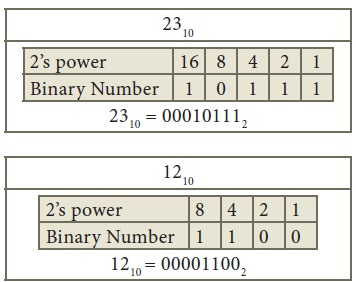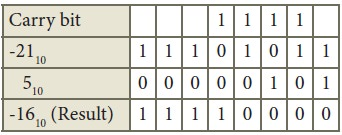Home | | Computer Technology 11th std | Binary Arithmetic

# Binary Arithmetic

1. Binary Addition 2. Binary Subtraction

Binary Arithmetic

As decimal numbers, the binary numbers also permit computations like addition, subtraction, multiplication and division. The following session deals only with binary addition and subtraction.

The following table is useful when adding two binary numbers.In 1 + 1 = 10, is considered as sum 0 and the 1 as carry bit. This carry bit is added with the previous position of the bit pattern.

Example Perform Binary addition for the following: 2310 + 1210

Step 1: Convert 23 and 12 into binary formStep 2: Binary addition of 23 and 12:## 2. Binary Subtraction

The table for Binary Subtraction is as follows:When subtracting 1 from 0, borrow 1 from the next Most Significant Bit, when borrowing from the next Most Significant Bit, if it is 1, replace it with 0. If the next Most Significant Bit is 0, you must borrow from a more significant bit that contains 1 and replace it with 0 and 0s upto that point become 1s.

Example Subtract 10010102 – 101002Example Perform binary addition for the following: (–21)10 + (5)10

Step 1: Change -21 and 5 into binary formStep 2:Step 3:

Binary Addition of –21 and 5 :Tags : Example, Step | Binary Addition and Subtraction , 11th Computer Science : Chapter 2 : Number Systems
Study Material, Lecturing Notes, Assignment, Reference, Wiki description explanation, brief detail
11th Computer Science : Chapter 2 : Number Systems : Binary Arithmetic | Example, Step | Binary Addition and Subtraction